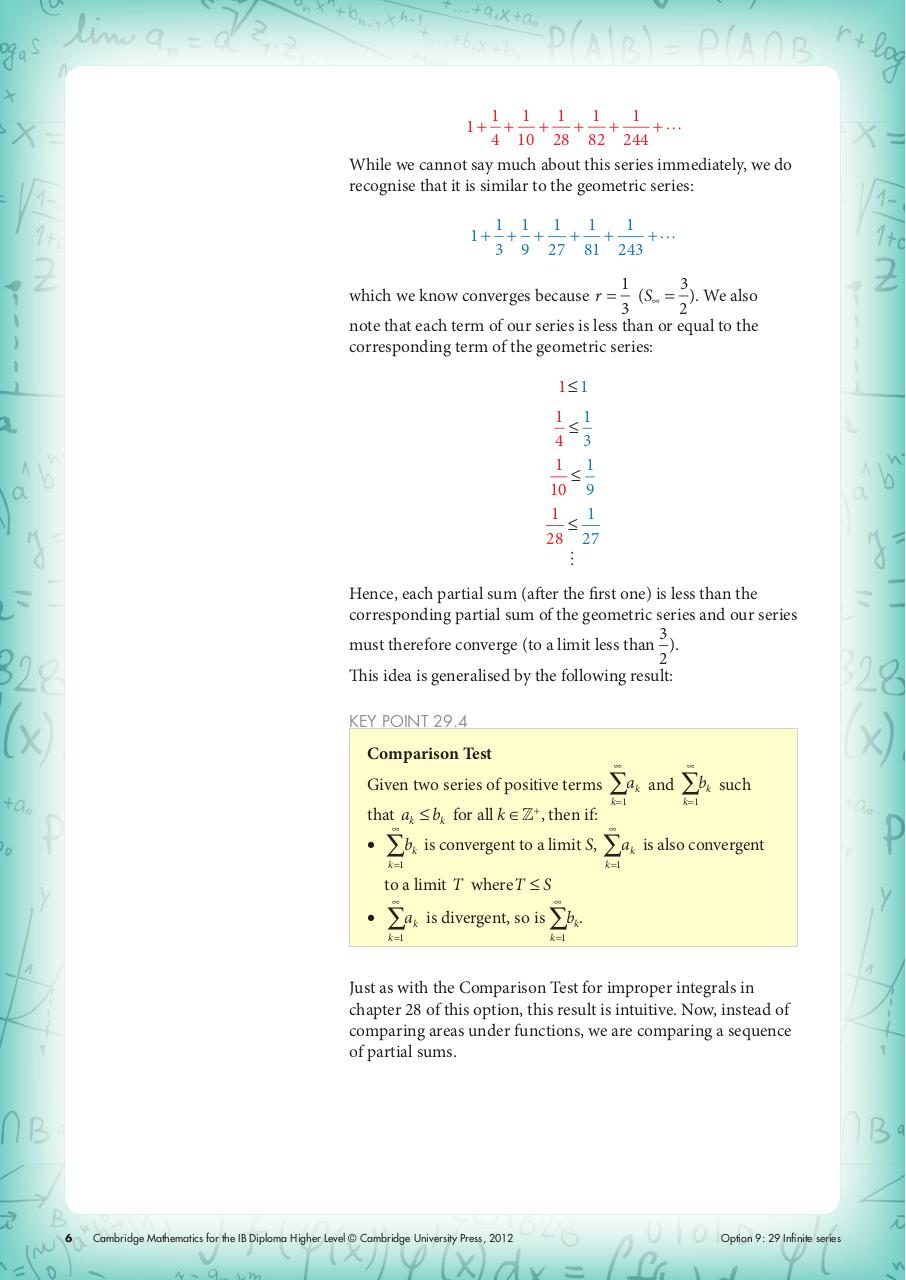# ch12.pdfPage 1 2 34526

#### Text preview

1 1
1
1
1 …
+ + + +
+
4 10 28 82 244
recognise that it is similar to the geometric series:
1+

1 1 1
1
1 …
1+ + + + +
+
3 9 27 81 243
1
3
(S∞ = ). We also
3
2
note that each term of our series is less than or equal to the
corresponding term of the geometric series:

which we know converges because r =

1≤1
1 1

4 3
1 1

10 9
1
1

28 27

Hence, each partial sum (after the first one) is less than the
corresponding partial sum of the geometric series and our series
3
must therefore converge (to a limit less than ).
2
This idea is generalised by the following result:
KEY POINT 29.4

Comparison Test

Given two series of positive terms
that ak

bk for all k ∈Z +, then if:

∑bk is convergent to a limit S,
k =1

to a limit T whereT

∑ak and
k =1

∑b

k

such

k =1

∑a

k

is also convergent

k =1

S

k =1

k =1

∑ak is divergent, so is ∑bk.

Just as with the Comparison Test for improper integrals in
chapter 28 of this option, this result is intuitive. Now, instead of
comparing areas under functions, we are comparing a sequence
of partial sums.

6

Cambridge Mathematics for the IB Diploma Higher Level © Cambridge University Press, 2012

Option 9: 29 Infinite series